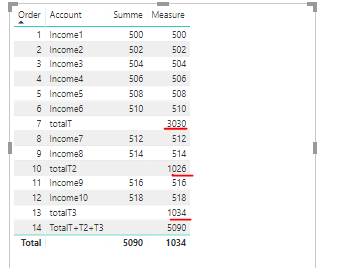cancel
Showing results for
Did you mean:Regular Visitor

## Financial Statement, Sum by Category

Hi,

I have a table with an account scheme like an income statement (see left table on the picture). I can create the values for each account for each month but not for the sum of those accounts in the same category. Furthermore I cannot implement calculated measures in the left table (like Total 1+ Total 2 + Total 3 = Total 4). On the right picture I calculated the totals which I want to implement in the table on the left. Do you have any ideas?For the values for each account I used: Balance = sumx(Testdaten;Testdaten[Summe])

For the sum by category (account) I used: SumbyCategory = CALCULATE(SUM(Testdaten[Summe]);ALLEXCEPT(Testschema;Testschema[Category]))

I also tried to use switch...but that doesn't show any data in the totals. Maybe I did something wrong there:

Switch =
IF (
COUNTROWS ( VALUES ( Testschema[Level]) ) = 1;
SWITCH (
VALUES ( 'Testschema'[Account] );
"Income 1"; [Balance];
"Income 2"; [Balance];
"Income 3"; [Balance];
"Income 4"; [Balance];
"Total T+T2+T3"; [Total4];

BLANK ()
);
100
)

I use a Date Table, a datatable and a table for the account scheme. Data looks like this:1 ACCEPTED SOLUTIONCommunity Support

Hi @Teletubbi ,

To create a measure as below.

```Measure =
VAR totalTid =
CALCULATE (
MAX ( 'Account'[Order] ),
FILTER ( ALL ( Account ), Account[Account] = "totalT" )
)
VAR totalt2id =
CALCULATE (
MAX ( 'Account'[Order] ),
FILTER ( ALL ( Account ), Account[Account] = "totalT2" )
)
VAR totalt3id =
CALCULATE (
MAX ( 'Account'[Order] ),
FILTER ( ALL ( Account ), Account[Account] = "totalT3" )
)
VAR totalid =
CALCULATE (
MAX ( 'Account'[Order] ),
FILTER ( ALL ( Account ), Account[Account] = "totalT+T2+T3" )
)
VAR _sum =
CALCULATE (
SUM ( 'Dataset'[Summe] ),
FILTER ( ALL ( Account ), 'Account'[Order] < totalTid )
)
VAR _sum2 =
CALCULATE (
SUM ( 'Dataset'[Summe] ),
FILTER ( ALL ( Account ), 'Account'[Order] <= totalt2id )
) - _sum
VAR sum3 =
CALCULATE (
SUM ( 'Dataset'[Summe] ),
FILTER ( ALL ( Account ), 'Account'[Order] <= totalt3id )
) - _sum - _sum2
VAR _all =
CALCULATE ( SUM ( 'Dataset'[Summe] ), ALL ( 'Dataset' ) )
RETURN
SWITCH (
TRUE (),
MAX ( Account[Account] ) = "totalT", _sum,
MAX ( Account[Account] ) = "totalT2", _sum2,
MAX ( Account[Account] ) = "totalT3", sum3,
MAX ( Account[Account] ) = "TotalT+T2+T3", _all,
ISBLANK ( CALCULATE ( SUM ( 'Dataset'[Summe] ) ) ) <> TRUE (), SUM ( 'Dataset'[Summe] )
)
```Pbix as attached.

Regards,

Frank

Community Support Team _ Frank
If this post helps, then please consider Accept it as the solution to help the others find it more quickly.
2 REPLIES 2Community Support

Hi @Teletubbi ,

To create a measure as below.

```Measure =
VAR totalTid =
CALCULATE (
MAX ( 'Account'[Order] ),
FILTER ( ALL ( Account ), Account[Account] = "totalT" )
)
VAR totalt2id =
CALCULATE (
MAX ( 'Account'[Order] ),
FILTER ( ALL ( Account ), Account[Account] = "totalT2" )
)
VAR totalt3id =
CALCULATE (
MAX ( 'Account'[Order] ),
FILTER ( ALL ( Account ), Account[Account] = "totalT3" )
)
VAR totalid =
CALCULATE (
MAX ( 'Account'[Order] ),
FILTER ( ALL ( Account ), Account[Account] = "totalT+T2+T3" )
)
VAR _sum =
CALCULATE (
SUM ( 'Dataset'[Summe] ),
FILTER ( ALL ( Account ), 'Account'[Order] < totalTid )
)
VAR _sum2 =
CALCULATE (
SUM ( 'Dataset'[Summe] ),
FILTER ( ALL ( Account ), 'Account'[Order] <= totalt2id )
) - _sum
VAR sum3 =
CALCULATE (
SUM ( 'Dataset'[Summe] ),
FILTER ( ALL ( Account ), 'Account'[Order] <= totalt3id )
) - _sum - _sum2
VAR _all =
CALCULATE ( SUM ( 'Dataset'[Summe] ), ALL ( 'Dataset' ) )
RETURN
SWITCH (
TRUE (),
MAX ( Account[Account] ) = "totalT", _sum,
MAX ( Account[Account] ) = "totalT2", _sum2,
MAX ( Account[Account] ) = "totalT3", sum3,
MAX ( Account[Account] ) = "TotalT+T2+T3", _all,
ISBLANK ( CALCULATE ( SUM ( 'Dataset'[Summe] ) ) ) <> TRUE (), SUM ( 'Dataset'[Summe] )
)
```Pbix as attached.

Regards,

Frank

Community Support Team _ Frank
If this post helps, then please consider Accept it as the solution to help the others find it more quickly.Community Support

Hi @Teletubbi ,

Does that make sense? If so, kindly mark my answer as the solution to close the case please. Thanks in advance.

Regards,
Frank

Community Support Team _ Frank
If this post helps, then please consider Accept it as the solution to help the others find it more quickly.